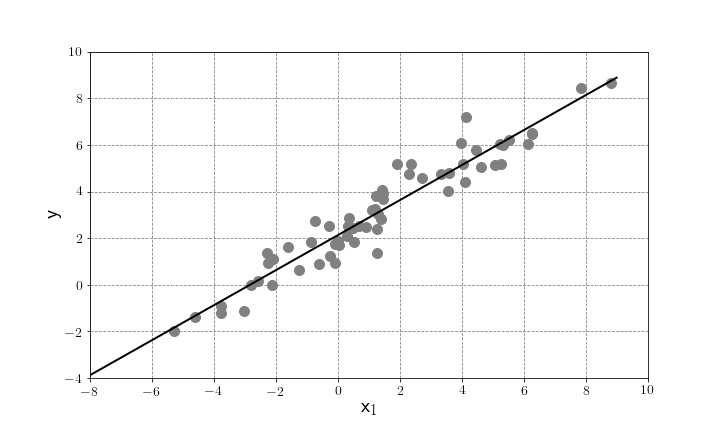# CoreML – Linear RegressionHello again, where were we? … Oh yes, we have been discussing CoreML and have even set up an appropriate python 2 environment to work with CoreML. In this post we are going to cover some of the most basic aspects of the workhorse of machine learning: the dependable linear regression model.

We are indeed all familiar with a line of best fit, and I am sure that many of us remember doing some by hand (you know who you are) and who hasn’t played with Excel’s capabilities? In a nutshell, a linear regression is a model that relates a variable$y$ to one or more explanatory (or independent) variables$X$. The parameters that define the model are estimated from the available data and there are a number of assumptions about the explanatory variables and you can find more information in my Data Science and Analytics with Python book. We can think of the goal of a linear regression model to draw a line though the data as exemplified in the plot below:Let us take the case of 2 independent variables$x_1$ and$x_2$. The linear regression model to predict our target variable$y$ is given by:$y=\alpha + \beta_1 x_1 + \beta_2 x_2 + \epsilon$,

where$\alpha$and$\beta_i$ are the parameters to be estimated to help us generate predictions. With the aid of techniques such as least squares can estimate the parameters$\alpha, \beta_1$ and$\beta_2$ by minimising the sum of the squares of the residuals, i,.e the difference between an observed value, and the fitted value provided by a model. Once we have determined the parameters, we are able to score new (unseen) data for$x_1$ and$x_2$ to predict the value of$y$.

In the next post we will show how we can do this for the Boston House Prices dataset using a couple of variables such as number of bedrooms in the property and a crime index for the area. Remember that the aim will be to show how to build the model to be used with CoreML and not a perfect model for the prediction.

Keep in touch.

-j

Also published on Medium.

## 3 thoughts on “CoreML – Linear Regression”

This site uses Akismet to reduce spam. Learn how your comment data is processed.